# KR101613829B1 - Method and Apparatus for 3D Shape Measuring By Using Derivative Moire - Google Patents

Method and Apparatus for 3D Shape Measuring By Using Derivative Moire Download PDF

## Info

Publication number
KR101613829B1
KR101613829B1 KR1020140055083A KR20140055083A KR101613829B1 KR 101613829 B1 KR101613829 B1 KR 101613829B1 KR 1020140055083 A KR1020140055083 A KR 1020140055083A KR 20140055083 A KR20140055083 A KR 20140055083A KR 101613829 B1 KR101613829 B1 KR 101613829B1
Authority
KR
South Korea
Prior art keywords
moire
absolute
phase
degree
φ
Prior art date
Application number
KR1020140055083A
Other languages
Korean (ko)
Other versions
KR20150129184A (en
Inventor
조춘식
배윤재
하병완
박지안
김주환
Original Assignee
조춘식
김주환
주식회사 화이버텍
Priority date (The priority date is an assumption and is not a legal conclusion. Google has not performed a legal analysis and makes no representation as to the accuracy of the date listed.)
Filing date
Publication date
Application filed by 조춘식, 김주환, 주식회사 화이버텍 filed Critical 조춘식
Priority to KR1020140055083A priority Critical patent/KR101613829B1/en
Publication of KR20150129184A publication Critical patent/KR20150129184A/en
Application granted granted Critical
Publication of KR101613829B1 publication Critical patent/KR101613829B1/en

## Images

•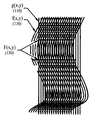•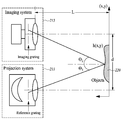•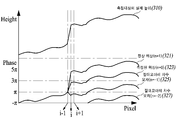•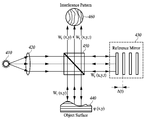••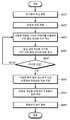••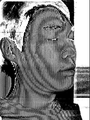•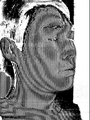•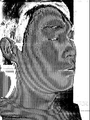•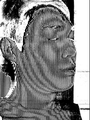•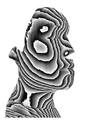•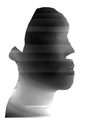•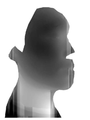•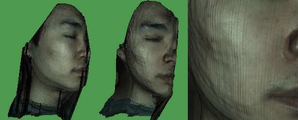## Classifications

• GPHYSICS
• G06COMPUTING; CALCULATING; COUNTING
• G06KRECOGNITION OF DATA; PRESENTATION OF DATA; RECORD CARRIERS; HANDLING RECORD CARRIERS
• G06K9/00Methods or arrangements for reading or recognising printed or written characters or for recognising patterns, e.g. fingerprints
• G06K9/36Image preprocessing, i.e. processing the image information without deciding about the identity of the image
• G06K9/46Extraction of features or characteristics of the image
• GPHYSICS
• G01MEASURING; TESTING
• G01BMEASURING LENGTH, THICKNESS OR SIMILAR LINEAR DIMENSIONS; MEASURING ANGLES; MEASURING AREAS; MEASURING IRREGULARITIES OF SURFACES OR CONTOURS
• G01B11/00Measuring arrangements characterised by the use of optical means
• G01B11/24Measuring arrangements characterised by the use of optical means for measuring contours or curvatures
• G01B11/25Measuring arrangements characterised by the use of optical means for measuring contours or curvatures by projecting a pattern, e.g. one or more lines, moiré fringes on the object
• GPHYSICS
• G01MEASURING; TESTING
• G01BMEASURING LENGTH, THICKNESS OR SIMILAR LINEAR DIMENSIONS; MEASURING ANGLES; MEASURING AREAS; MEASURING IRREGULARITIES OF SURFACES OR CONTOURS
• G01B11/00Measuring arrangements characterised by the use of optical means
• G01B11/24Measuring arrangements characterised by the use of optical means for measuring contours or curvatures
• G01B11/25Measuring arrangements characterised by the use of optical means for measuring contours or curvatures by projecting a pattern, e.g. one or more lines, moiré fringes on the object
• G01B11/254Projection of a pattern, viewing through a pattern, e.g. moiré
• G02B30/00
• GPHYSICS
• G06COMPUTING; CALCULATING; COUNTING
• G06KRECOGNITION OF DATA; PRESENTATION OF DATA; RECORD CARRIERS; HANDLING RECORD CARRIERS
• G06K9/00Methods or arrangements for reading or recognising printed or written characters or for recognising patterns, e.g. fingerprints
• G06K9/00201Recognising three-dimensional objects, e.g. using range or tactile information
• GPHYSICS
• G06COMPUTING; CALCULATING; COUNTING
• G06KRECOGNITION OF DATA; PRESENTATION OF DATA; RECORD CARRIERS; HANDLING RECORD CARRIERS
• G06K9/00Methods or arrangements for reading or recognising printed or written characters or for recognising patterns, e.g. fingerprints
• G06K9/20Image acquisition
• G06K9/2036Special illumination such as grating, reflections, deflections, e.g. for characters with relief
• GPHYSICS
• G06COMPUTING; CALCULATING; COUNTING
• G06TIMAGE DATA PROCESSING OR GENERATION, IN GENERAL
• G06T7/00Image analysis

## Abstract

A three-dimensional shape measuring method and apparatus using differential Moiré are disclosed.
According to an aspect of the present embodiment, there is provided a three-dimensional shape measuring apparatus using a moire interference method, comprising: a unit corrector for obtaining a relative correction value of an absolute moire degree by using a phase difference between two adjacent measurement points; And a phase correcting unit for correcting the phase of the target point by using the absolute moire degree, and a phase correcting unit for correcting the phase of the target point by using the absolute moire degree, Dimensional shape measuring apparatus.

## Description

BACKGROUND OF THE INVENTION 1. Field of the Invention The present invention relates to a three-dimensional shape measurement method and apparatus using a differential moire,

The present invention relates to a method and an apparatus for measuring a three-dimensional shape using a differential moire, and more particularly to an improved measuring method and apparatus for overcoming 2π ambiguity in a moire interference method.

The contents described in this section merely provide background information on the present embodiment and do not constitute the prior art.

The 3D shape measurement method is a technology field that has received much attention in recent years. The three-dimensional shape measurement technology is remarkably developed due to the expansion of the industrial application range such as medical, manufacturing, and biometrics.

Representative three-dimensional shape measurement methods include laser scanning, time of flight (TOF), stereo vision, moire interference, and the like.

Laser scanning is a method of acquiring three-dimensional information of an object by emulating a laser on the object and observing the shape change of the laser seen from the side. Precise three-dimensional information can be obtained, but the measurement speed is slow because a laser or an object must be moved.

Time of Flight is a method of acquiring three-dimensional information of an object by emulating Pulsed Light or RF Modulated Light on an object, then comparing the emitted light with the reflected light . The Pulsed Light technique is a method of calculating the distance by measuring the time from when the light emitted from the emitter is reflected on the object to returning. The RF Modulated Light technique measures the phase shift of the light reflected from the object It is a way to calculate the distance. Although the measurement speed is fast, the light is distorted or scattered while moving between the emitter and the object, and the accuracy is lowered.

Stereo Vision is the application of the principle that a human being feels stereoscopic effect of an object by using two eyes. It shoots the same object using two cameras and then compares two images and obtains three-dimensional information of the object . Although it is possible to acquire three-dimensional information of an object by a relatively inexpensive and simple method, it is difficult to measure an object at a distance and an algorithm for finding a matching point between two images is complicated.

Therefore, the three-dimensional shape measurement method which is currently attracting attention is Moire Interference. Moire interferometry is a method of observing the moire that occurs when a grating with a constant spacing (Grid) over an object overlaps a deformed grating and a reference grating on an object Dimensional information of an object.

Using Moire interferometry, it is possible to perform 3D shape measurement faster and more efficiently than other measurement methods. Nevertheless, the lack of application of moire interferometry in real industry is due to two limitations of moire interferometry.

The first limitation is that the phase acquired by the moire interferometry is not clear due to noise, and the second limit is the reliability of the phase unwrapping due to a measurement error called 2? Ambiguity.

For the first limit, noise can be removed using a cosine transform, a wavelet transform, or the like. However, there is no clear solution to the second limitation. Although a method of solving the problem of 2? Ambiguity has been proposed by using a Least Square Method, the least squares method has a problem in that calculation is complicated and the sudden phase change is not properly reflected.

An object of the present invention is to provide a method and apparatus for measuring a three-dimensional shape using Moire interferometry, which can solve a problem of 2? Ambiguity more quickly and accurately than a conventional method.

According to an aspect of the present embodiment, in a three-dimensional shape measuring apparatus using a moire interference method, relative phase correction of an absolute moire order is performed using a phase difference between two adjacent measuring points, An adjust unit for obtaining an adjust value; An integrated correction unit for correcting an absolute moire degree using the correction value to a target point and a phase adjusting unit for correcting the phase of the target point using the absolute moire degree, The present invention provides a 3D Shape Measuring Apparatus using a moire interference method, which includes an adjust unit.

According to another aspect of the present invention, in a three-dimensional shape measuring method using a moire interference method, a relative correction value of an absolute moire degree is calculated by using a phase difference between two adjacent measurement points A unit calibration process to obtain; And a phase correction step of correcting the phase of the target point by using the absolute moire degree. The method of claim 1, wherein the phase correction step comprises: And provides a 3D Shape Measuring Method.

According to this embodiment, in the three-dimensional shape measurement using the moire interference method, accurate height measurement can be performed even if the height change between adjacent two measurement points is abrupt, thereby improving the reliability of the three-dimensional shape measurement using the moire interference method It is possible to extend the application range of the moire interference method throughout the industry.

FIG. 1 is a view showing a moire pattern. FIG.
FIG. 2 is a diagram showing a measurement principle of a projection moire interference method.
FIG. 3 is a diagram showing the 2? Ambiguity of the moire interference method. FIG.
4 is a diagram showing the principle of measurement of a phase shifting moire interference.
5 is a block diagram of a three-dimensional shape measuring apparatus using a derivative moire.
6 is a flowchart of a method of measuring a three-dimensional shape using a derivative moire.
7 is a face of a person to be measured in an experiment for verifying the present embodiment.
8 to 11 are deformed gratings obtained by phase shifting moire interference in an experiment for verifying the present embodiment.
12 is a moire pattern generated when a deformed grating obtained by a phase shifting moire interference is imaged on a reference grating in an experiment to verify the present embodiment.
FIG. 13 is a result of differentiating the moire pattern generated when the deformed grating acquired by the phase shifting Moire Interference is imaged on the reference grating in the x-axis direction in the experiment for verifying the present embodiment.
FIG. 14 is a result of differentiating the moire pattern generated when the deformed grating acquired by the Phase Shifting Moire Interference is imaged on the reference grating in the y-axis direction in the experiment for verifying the present embodiment.
FIG. 15 is a final three-dimensional shape obtained by using an absolute moire degree calculated using a derivative moire in an experiment for verifying the present embodiment.

Hereinafter, some embodiments of the present invention will be described in detail with reference to the accompanying drawings. It should be noted that, in the drawings, like reference numerals are used to denote like elements in the drawings, even if they are shown in different drawings. In the following description of the present invention, detailed description of known functions and configurations incorporated herein will be omitted when it may make the subject matter of the present disclosure rather unclear.

In describing the constituent elements of this embodiment, first, second, i), ii), a), b) and the like can be used. Such a code is intended to distinguish the constituent element from other constituent elements, and the nature of the constituent element, the order or the order of the constituent element is not limited by the code. It is also to be understood that when an element is referred to as being "comprising" or "comprising", it should be understood that it does not exclude other elements unless explicitly stated to the contrary, do. Also, the terms 'module', 'module' and the like described in the specification mean a unit for processing at least one function or operation, which can be implemented as 'hardware' or 'software' or 'combination of hardware and software' have.

This embodiment relates to a measurement method and apparatus for overcoming 2? Ambiguity necessarily occurring in the moire interference method.

2π ambiguity is a problem caused by the fact that the moire pattern has a period of the cosine function. Due to the ambiguity of 2π, it is impossible to acquire accurate three-dimensional information by only information provided by the moire pattern.

So far, many ways to overcome 2π ambiguity have been proposed, but it is either too much or too complicated to completely overcome 2π ambiguity. The present embodiment provides a measurement method and apparatus for solving the problem of 2? Ambiguity simply and efficiently by differentiating the phase of moire pattern unlike the existing methods.

Since the present embodiment uses differential calculations, the measurement method and apparatus according to the present embodiment is referred to as a measurement method and apparatus using differential Moire.

In order to understand the problem-solving principle of this embodiment, it is necessary to understand 2π ambiguity. I) Moire Interference, ii) Projection Moire Interference, iii) 2π ambiguity of projection moire interferometry, iv) Phase Shifting Moire Interference, v. ) Phase shifting After briefly mentioning the 2? Ambiguity of the moire interference method, the differential moire will be described in detail.

1. moire interferometry

Moire Pattern is a pattern that occurs when overlapping patterns such as overlapping silk or overlapping mosquito nets overlap. When several patterns with similar cycles are overlapped, a beating effect generates a unique pattern at a low frequency, which is called a moire pattern.

The moire pattern has various qualities. Among them, engineers note that the moire pattern can amplify the motion of the object considerably and that the moire pattern has three-dimensional information about the shape of the object. By using this property, it becomes possible to analyze the micro-behavior of the object or measure the three-dimensional shape of the object.

An interference fringe is generated when two light sources that start at the same reference point travel in different paths of light. Since the interference pattern generated at this time is due to the difference in light path, if the interval of the interference fringes is known, the distance from the light source to the interference fringe can be calculated inversely. A moire pattern is also a kind of interference pattern, and the height from the light source to the surface of the object can be obtained in a similar way.

The moire pattern is acquired by the moire interference method. The moire interference method is divided into a shadow moire interference method and a projection moire interference method.

2. Projection moire interference method

The projected moire interferometry is a method of observing a moire pattern generated when a grating pattern is projected on an object to be measured and then a deformed grating and a reference grating are overlapped on the object to be measured. Projection moire interferometry allows measurement of large objects over long distances. Therefore, the method generally used in the moire interference method is a projection type, and this embodiment also uses a projection moire interference method.

In order to measure the three-dimensional shape of an object by projection-type moire interferometry, the reference grating must first be projected onto the object. The projected grating is deformed according to the shape of the object. When a deformed grating is imaged on a reference grating, a moire pattern appears. The Moire pattern that appears at this time looks like a contour line, so you can use it to grasp the flatness of an object.

1 is a view showing a moire pattern.

In FIG. 1, when a reference grating 110 having a vertical shape is projected on an object, the grating is deformed according to the bending of the object at the surface of the object (120). If the deformed grating 120 is imaged on the reference grating 110, there is a horizontal stripe that did not exist before the image formation, which is the moire pattern 130. [

2 is a diagram showing the measurement principle of the projection type moire interference method.

And projects a reference grating toward the surface of the object 220 in a projection system 211. The reference grating is deformed on the surface of the object 220 and the imaging grating is imaged on a reference grating in an imaging system 213 to form a moiré pattern.

3. 2π ambiguity of projection-type moire interferometry

When a grating with a pitch G is projected by a projection system with a magnification of M, the deformed grating at the measurement point x of the surface of the object can be expressed as in Equation 1 Light intensity.

In Equation 1, I 1 (x, y) is the intensity of light of the modified grating, I source is the intensity of light source, R is the reflectivity of the surface of the object, A is the modulation of the grating (x, y) is the height of the measuring point, θ 1 is the angle of the projection system with respect to the measuring point, M is the magnification of the projection system, G Represents the pitch of the grating.

The deformed grating is reflected on the surface of the object and is then imaged on the reference grating of the imaging system to form a moire pattern. At this time, it can be assumed that the reference grating is projected from the imaging system to the surface of the object for simple analysis. In this case, the reference grating has the light intensity as shown in Equation 2 on the surface of the object.

In Equation 2 I 2 (x, y) is the light intensity of the reference grating, A is the modulation of the grating, r (x, y) is the distance of the measurement point, h (x, y) is height of measurement point, θ 2 is the measurement point M represents the magnification of the projection system, and G represents the pitch of the grating.

An image formed by imaging a deformed grating on a reference grating has a light intensity as shown in Equation (3).

In Equation 3, I (x, y) represents the light intensity of the image on the image, I 1 (x, y) represents the light intensity of the modified grating, and I 2 (x, y) represents the light intensity of the reference grating.

Expansion of equation (3) leads to equation (4).

In the equation (4), I (x, y) is the intensity of the image on the image, I source is the intensity of the light source , R is the reflectivity of the surface of the object, A is the modulation of the grating, r (x, y) is the height of the measuring point, θ 1 is the angle of the projection system relative to the measuring point, θ 2 is the angle of the imaging system with respect to the measuring point, M is the magnification of the projection system and G is the pitch of the grating.

In Equation (4), the first term represents the background image of the entire image, the second term represents the image of the modified grating, the third term represents the image of the reference grating, and the fourth term represents the interference image of the two gratings.

Expression 5 is the sum of the term corresponding to the moire pattern in the expanded mathematical expression after developing the fourth term.

I moire (x, y) is the light intensity on the image formation, I source is the intensity of the light source, R is the surface of the object reflectivity, A is the modulation of the grating, h (x, y) is the height of the measurement point in the equation (5), θ 1 is the angle of the projection system relative to the measuring point, θ 2 is the angle of the imaging system with respect to the measuring point, M is the magnification of the projection system, and G is the pitch of the grating.

As can be seen from Equation (5), the moire pattern has a period by the cosine function. At this time, the phase Φ of the moire pattern is expressed by Equation (6).

H (x, y) is the height of the measurement point,? 1 is the angle of the projection system relative to the measurement point,? 2 is the phase of the imaging system for the measurement point Angle, M is the magnification of the projection system, and G is the pitch of the grating.

Applying a trigonometric function to the horizontal distance from the projection system to the surface of the object, Equation (7) holds.

In equation (7), θ 1 is the angle of the projection system with respect to the measuring point, θ 2 is the angle of the imaging system with respect to the measuring point, d is the distance between the projection system and the imaging system, L is the distance from the projection system to the reference plane, h , y) represents the height of the measurement point.

When Equation (6) and Equation (7) are combined, Equation (8) is derived.

(X, y) is the phase of the moire pattern at the measurement point, d is the spacing between the projection system and the imaging system, M is the magnification of the projection system, G is the pitch of the grating, L Is the distance from the projection system to the baseline, and h (x, y) is the height of the measurement point.

Since the moire pattern is represented by the cosine function, the phase of the moire pattern is repeated with a period of 2π. Hence, Equation (8) should be exactly expressed as Equation (9).

In equation (9), Φ 0 is the current phase of the moire pattern, n is the absolute moire order, d is the spacing between the projection system and the imaging system, M is the magnification of the projection system, G is the pitch of the grating, The distance from the projection system to the reference plane, h (x, y), represents the height of the measurement point.

The absolute moire degree n is information on how many 2π cycles the current phase? 0 of the moire pattern has undergone. Knowing the current phase Φ 0 of the moire pattern does not allow accurate height measurement, so you need to know the absolute moire degree n of the measurement point.

If the absolute moire degree n of the measurement point is not known, the phase is calculated in a relative manner using the phase difference between two adjacent measurement points.

If the two measurement points are very close, it can be assumed that the phase difference between the two measurement points is less than 2 ?. If the phase difference between two adjacent measurement points is smaller than 2π, the phases of adjacent measurement points can be calculated by adding the phase difference from the measurement point adjacent to the measurement point whose phase is known. Calculating the phase of a measurement point in this way is called phase unwrapping.

However, since the phase expansion is based on the assumption that the phase change between two adjacent measurement points is smaller than 2π, a measurement error occurs when the phase change between two adjacent measurement points exceeds 2π. This is called 2π ambiguity.

3 is a diagram showing 2? Ambiguity in the moire interference method.

3, the height 310 of the measurement object suddenly increases between two adjacent measurement points i-1 and i. As a result, the phase shift of the moire pattern between measurement points i-1 and i exceeds 2 ?. Since the phase change exceeding 2? Can not be grasped only by the moire pattern, the height (323) of the measuring point calculated by only the moire pattern is different from the actual one.

In this case, the phase of the measurement point i must be corrected using the absolute moire degree n. In Fig. 4, the absolute moire degree for correcting the phase of the measurement point i is n = 1. Therefore, when n = 1 is applied, a correct phase 321 can be obtained.

Incorrect calculation of the absolute moire order results in a false phase. For example, it can be seen that the phase 327 obtained by applying n = -1 or the phase 327 obtained by applying n = -2 does not properly reflect the actual height of the measurement object.

If the absolute moire degree n is known, the correct phase will be obtained even if the phase change between two adjacent measurement points exceeds 2π. However, it is not easy to obtain the absolute moire degree n, and an accurate solution is not yet presented.

4. Phase shifting moire interferometry

In the phase shifting moire interferometer, which is widely used in recent years, 2? Ambiguity is a problem.

The general Moiré interference method is not suitable for precise measurement because of its low resolution. In order to increase the resolution of the Moire interferometry, a phase shifter moire interference technique is developed.

The phase shifting moire interferometry is a method of acquiring a plurality of interference signals while moving a reference mirror with a fine actuator and obtaining the shape and the height of the interference signal at each measurement point in the image And is a way of interpreting the mathematical relationship with. Phase shifting moire interferometry is widely used because of its high resolution and its ability to measure three-dimensional shape irrespective of the shape of the moire pattern.

4 is a diagram showing the measurement principle of the phase-shifting moire interference method.

First, light (mainly white light) is emitted from the light source 410 toward the condenser 420. The light uniformized through the condenser lens reaches the reference mirror 430 and the surface of the measurement object 440 via a spectrometer 450. The light reflected from the reference mirror 430 and the light reflected from the surface of the measurement object 440 reach the imaging system again via the spectroscope 450 and form the moire pattern 460 through the imaging.

In the phase shifter moire interference method, the reference mirror 430 is moved by the fine driver. When the reference mirror 430 moves, a phase difference occurs due to an optical path difference. In the commonly used 4-Bucket Algorithm, the light intensity obtained when the phase difference is 0, 90, 180, or 270 degrees is acquired, and three-dimensional information of the measurement object is obtained by analyzing the light intensity.

5. 2π ambiguity of phase shifting moire interferometry

In the phase shifting moire interferometry, the relationship between the height of the measurement point, the phase of the measurement point, and the length of the equivalent wavelength to be projected is shown in Equation (10).

In Equation (10), h (x, y) is the height of the measurement point, λ eq is the length of the equivalent wavelength, and Φ (x, y) indicates the phase of the moire pattern at the measurement point.

In the phase shifting moire interferometry, the phase of the moire pattern appearing at the measurement point P (x, y) is expressed by the following equation (11).

In Equation (11),? 0 represents the current phase of the moire pattern. In the phase-shifting moire interferometry, the light intensities when the phase differences between the two gratings are sequentially 0, 90, 180, and 270 degrees through phase shift are denoted by I 1 ( (x, y), I 2 (x, y), I 3 (x, y), and I 4 (x, y).

Since the moire pattern is represented by the cosine function, the phase of the moire pattern is repeated with a period of 2π. However, since the phase of the moiré pattern obtained through the phase shifting moire interference method is represented by an arc tangent and the arc tangent has only a value from -π / 2 to π / 2, The phase that can be grasped by the transplant moiré interference method is only from -π / 2 to π / 2. Therefore, in the case of using the phase shifting moire interferometry, the problem of 2? Ambiguity occurs when the slope between adjacent two measurement points exceeds?. Specifically, if π is substituted in Equation 10, it can be seen that a problem of 2π ambiguity occurs when the height difference between two adjacent measurement points exceeds λ eq / 4.

Referring to Equation (10), when the length of the equivalent wavelength lambda eq is large, the height difference between two measurable adjacent points becomes large. That is, 2π ambiguity can be prevented by increasing the length λ eq of the equivalent wavelength. However, the larger the length of the equivalent wavelength λ eq , the lower the resolution.

According to Equation (11), the phases that can be distinguished in the phase shifter moire interferometry range from -π / 2 to? / 2 by?. Therefore, in the phase shifting moire interference method, the phase of the moire pattern must be exactly expressed as: &quot; (12) &quot;

In Equation (12),? (X, y) represents the phase of the moire pattern at the measurement point,? 0 represents the current phase of the moire pattern, and n represents the absolute moire degree.

6. Differential moiré technique

The differential Moiré scheme proposed in this embodiment solves the problem of 2? Ambiguity by grasping the phase in which the absolute moire degree changes between two adjacent measurement points by differentiating the phase of the moire pattern and obtaining the absolute moire degree through it.

This embodiment solves the problem of 2? Ambiguity by distinguishing the case where the absolute moire degree rises between the two adjacent measurement points, the case where the absolute moire degree increases, and the case where it does not change.

A specific method of classification is shown in Equation (13).

The function u (t) in equation (13) is a function with u (t) = 1 when t? 0 and u (t) = 0 otherwise. Φ 0 is the current phase of the moiré pattern, and Φ ddiscriminant ) is a constant number to distinguish between cases where the absolute moire order varies between two adjacent measurement points.

Using Equation (13), a correction value of the absolute moire degree for the current measurement point can be obtained, which is referred to as a unit absolute moire degree. The absolute moire degree for the x-axis can be calculated by applying Equation (13) successively to adjacent measurement points.

When the target point to be measured is P (i, j), the variation of the absolute moire degree when the x-axis coordinate is 1 and the variation of the absolute moire degree when the x-axis coordinate is 2 after fixing the y- And ... If the variation of the absolute moire degree is continuously added when the x-axis coordinate is i, a correction value of the absolute moire degree at the target point can be obtained, which is referred to as an integrated absolute moire degree for the x-axis. This can be expressed by the following equation (14).

The function u (t) in equation (14) is a function with u (t) = 1 when t? 0 and u (t) = 0 otherwise. Φ ddiscriminant ) is a constant for distinguishing between the case where the absolute moire degree is changed between the adjacent two measurement points and the case where the absolute moire degree is not changed between the adjacent two measurement points , n i, j is the integrated absolute moire degree for the x axis, Φ 0 is the current phase of the moire pattern, .

In Equation (14), the value of? D may vary depending on the measurement method and the measurement object, and the value of? D for obtaining a correct result is determined experimentally. The experiment for obtaining the value of? D can be easily performed by a person having ordinary skill in the art to which the present invention belongs.

In general, Φ d is selected to be extremely close to π among the values larger than 0 and smaller than π. For example, in experiments with human faces and plaster casts, the most accurate results were obtained when φ d was 0.8π.

The value of Equation (14) can be calculated through Equation (15).

Φ 0 is the current phase of the Moiré pattern in the equation 15, I 1 (x, y ), I 2 (x, y), I 3 (x, y), I 4 (x, y) is a phase shifter transplantation moire interference And the phase difference between the two gratings is sequentially 0, 90, 180, and 270 degrees.

the phase unwrapping is performed in the x-axis direction at the measurement point P (i, j) using the absolute moire degree for the x-axis (see Equation 16).

In the equation (16), 陸i, j x represents a phase in which phase expansion is completed for the x axis, 陸0 represents a current phase of the moire pattern, and n i, j represents an integrated absolute moire degree for the x axis.

In order to obtain the absolute moire degree by differentiating the moire pattern and correct the phase through it, not only the x-axis direction but also the y-axis direction should be considered. This is because there is no abrupt gradient change along the horizontal axis (x axis) depending on the shape of the object, and a sudden gradient change occurs on the vertical axis (y axis) in many cases. For example, in the case of the nose and the jaw in the face of a person, the change in height to the horizontal axis is small, but the change in height to the vertical axis is large. In this case, if the phase expansion is performed only in the x-axis direction, the correct phase can not be obtained. Therefore, the phase expansion should also be performed in the y-axis direction.

The absolute moire degree for the y-axis is calculated as follows.

When the target point to be measured is P (i, j), the variation of the absolute moire degree when the y axis coordinate is 1 and the variation of the absolute moire degree when the y axis coordinate is 2 after fixing the x axis coordinate to i And ... If the variation of the absolute moire degree is continuously added when the y-axis coordinate is j, a correction value of the absolute moire degree at the target point can be obtained, which is referred to as an integrated absolute moire degree for the y-axis. This can be expressed by the following equation (17).

In equation (17), the function u (t) is a function with u (t) = 1 when t? 0 and u (t) = 0 otherwise. Φ ddiscriminant ) is a constant for distinguishing between the case where the absolute moire degree changes between the adjacent two measurement points and the case where the absolute moire degree does not change between the adjacent two measurement points , n i, j x is the absolute moire degree for the y axis, Φ 0 is the current phase of the moire pattern, .

The value of Equation (17) can be calculated through Equation (18).

Φ 0 is the current phase of the Moiré pattern in the equation 18, I 1 (x, y ), I 2 (x, y), I 3 (x, y), I 4 (x, y) is a phase shifter transplantation moire interference And the phase difference between the two gratings is sequentially 0, 90, 180, and 270 degrees.

(see Equation 19) using the absolute moire degree for the y-axis at the measurement point P (i, j) in the y-axis direction.

In the equation (19), 陸i, j is a phase in which phase expansion is completed with respect to the x and y axes, 陸i, j x is a phase in which phase expansion is completed with respect to the x axis, n i and j x are absolute moirés Represents the order.

Since the obtained phase is an accurate phase without the problem of 2? Ambiguity, the accurate height of the measurement point can be obtained by using this phase (see Equation 10).

7. 3D shape measuring device using differential Moiré technique

5 is a block diagram of a three-dimensional shape measuring apparatus using differential Moiré.

The three-dimensional shape measuring apparatus using differential Moiré solves the problem of 2? Ambiguity by adding the differential moire calculating unit 500 between the phase measuring unit 550 and the phase output unit 570 of the moire measuring apparatus.

The differential Moiré operation unit 500 includes a unit correction unit 510, an integrated correction unit 520, and a phase correction unit 530.

The unit correction unit 510 includes a unit derivative unit 511. The unit differential section 511 performs a function of differentiating the phase of the moire pattern.

The unit differential section 511 includes a unit absolute moire degree calculator 513. The unit absolute moire degree calculator 513 determines the degree by which the absolute moire degree changes relatively using Equation (13).

The integrated correction unit 520 sums the unit absolute moire degree.

The integrated correction unit 520 includes a first correction unit 521. The first calibration unit 521 sums the unit absolute moire degree with respect to the x-axis.

The first correction unit 521 includes a first calculation unit 523. The first calculation unit 523 calculates an integrated absolute moire degree for the x-axis using Equation (14).

The integrated correction unit 520 also includes a second correction unit 521. [ The second calibration unit 525 sums the unit absolute moire degree with respect to the y-axis.

The second correction unit 525 includes a second calculation unit 527. [ The second arithmetic operation unit 527 obtains the integrated absolute moire degree for the y-axis by using the equation (17).

The phase corrector 530 corrects the phase of the target point using the finally obtained integrated absolute moire degree.

Since the obtained phase is an accurate phase without the problem of 2? Ambiguity, the accurate height of the measurement point can be obtained by using this phase (see Equation 10).

8. 3D shape measurement method using differential Moiré technique

6 is a flowchart of a method of measuring a three-dimensional shape using differential moire.

Dimensional shape measurement method using differential moiré solves the problem of 2? Ambiguity by adding the differential moiré calculation process (S620 to S670) between the phase measurement step S610 of the target point and the height output step S680 of the target point.

The phase of the starting point is measured for the differential moiré calculation (S620).

A unit absolute moire degree between the adjacent measurement points starting from the origin is obtained (S630).

The integrated absolute moire degree is calculated by summing the unit absolute moire degree between adjacent measurement points until the target point is reached (S640).

When the target point is reached, the summing is stopped (S650), and the phase of the target point is corrected using the finally obtained integrated absolute moire degree (S660).

Since the obtained phase is an accurate phase without the problem of 2? Ambiguity, the accurate height of the measurement point can be obtained by using this phase (see Equation 10).

9. Verification of differential Moiré technique

Hereinafter, it is verified that the problem of 2? Ambiguity is solved by using differential Moiré.

7 is a face of a person to be measured in an experiment for verifying the present embodiment.

The face of the person to be measured was separated by 60 cm from the projection system. The projection system projects a reference grating with a 1 mm gap and a size of 28 cm x 35 cm towards the object being measured.

Figs. 8 to 11 are modified gratings acquired by the phase shift method in the experiment for verifying the present embodiment.

In the phase shifting moire interferometry, the deformed lattices are shown in order when the phase difference between the two gratings is changed to 0 °, 90 °, 180 ° and 270 ° through phase shift.

Fig. 12 is a moire pattern obtained when an image obtained by phase-shifting a deformed grating is imaged on a reference grating in an experiment to verify the present embodiment.

It can be seen that the 3-dimensional information of the measurement object can not be acquired normally due to the 2? Ambiguity.

13 is a result of differentiating the moire pattern generated when the deformed grating acquired by the phase shift method is imaged on the reference grating in the x-axis direction in the experiment for verifying the present embodiment.

14 is a result of differentiating the moire pattern generated when the deformed grating acquired by the phase shift method is imaged on the reference grating in the y axis direction in the experiment to verify the present embodiment.

15 is a final three-dimensional shape obtained by using the absolute moire degree calculated using the differential moiré in the experiment for verifying the present embodiment.

It can be seen that the use of the differential Moiré can accurately acquire the three-dimensional information of the measurement object without the problem of 2? Ambiguity.

The present embodiment applies differential phase moiré to the phase-shifting moire interferometry. However, those skilled in the art will appreciate that the phase-shifting moire interferometry as well as the projection moire interferometry, It is possible to solve the problem of 2? Ambiguity by using the method or apparatus of this embodiment also for the interference method and other kinds of moire interference methods.

It will be apparent to those skilled in the art that various modifications and variations can be made in the present invention without departing from the spirit and scope of the invention as defined by the appended claims. It will be possible. The present invention is not intended to limit the scope of the present invention but to limit the scope of the present invention. The scope of protection of the present invention should be construed according to the claims, and all technical ideas considered to be equivalent or equivalent thereto should be construed as being included in the scope of the present invention.

110: reference grid 120: deformed grid
130: moire pattern 211: projection system
213: image forming system 220: measurement object
310: actual height of the measurement object 321: phase when n = 1
323: phase when n = 0 325: phase when n = -1
327: phase when n = -2 410: light source
420: condenser lens 430: reference mirror
440: object to be measured 450: spectroscope
460: moire pattern 500: differential moire calculator
510: unit correction unit 511: unit differential unit
513: unit absolute moire degree calculation unit 520:
521: first correction unit 523: first calculation unit
525: second correction unit 527: second operation unit
530: phase correction unit 550: phase measurement unit
570: phase output section

## Claims (11)

1. A three-dimensional shape measuring apparatus using a moire interference method,
A unit corrector for obtaining a relative correction value of an absolute moire degree by using a phase difference between two adjacent measurement points;
An integral corrector for correcting an absolute moire degree by using the correction value to a target point; And
And a phase correcting unit for correcting the phase of the target point using the absolute moire degree,
Wherein the unit correction unit differentiates the phase of the measurement point on the side closer to the origin of the two measurement points to obtain the relative correction value,
Dimensional shape measuring device using a moire interference method.
2. delete
3. The method according to claim 1,
Wherein the unit differential section comprises:

(t) is a function with u (t) = 1 when t ≥ 0 and u (t) = 0 otherwise Φ 0 is the current phase of the moiré pattern Φ ddiscriminant ) A constant for dividing the case where the absolute moire degree varies between a case where the absolute moire degree is changed and a case where the absolute moire degree is not changed)
Dimensional shape measuring device using a moire interference method.
4. The method according to claim 1,
The integrated correction unit may include:
a first correcting unit for correcting the absolute moire degree for the x axis; And
a second correction unit for correcting the absolute moire degree for the y-
Dimensional shape measuring device using a moire interference method.
5. 5. The method of claim 4,
The first correction unit

(t) is a function with u (t) = 1 when t≥0 and otherwise u (t) = 0. n i, j is the absolute moiré order for the x axis, Φ 0 is the moiré pattern The present phase Φ ddiscriminant ) includes a first arithmetic unit for calculating a constant for distinguishing between the case where the absolute moire degree changes between the two adjacent measurement points and the case where the absolute moire degree is not changed,
The second correction unit

(t) is a function with u (t) = 1 when t ≥ 0 and otherwise u (t) = 0. n i, j x is the absolute moiré order for the y axis Φ 0 is the moiré pattern The second phase angle Φ ddiscriminant ) is a constant for distinguishing between the case where the absolute moire degree varies between two adjacent measurement points and the case where the absolute moire degree is not changed)
Dimensional shape measuring device using a moire interference method.
6. In the three-dimensional shape measuring method using the moire interference method,
A unit derivative process of obtaining a relative correction value of an absolute moire degree by differentiating a phase of a measurement point near the origin among two adjacent measurement points;
An integrated correction process for correcting an absolute moire degree by using the correction value until reaching a target point; And
A phase correction process for correcting the phase of the target point using the absolute moire degree;
Dimensional shape measuring method using a moire interference method.
7. delete
8. The method according to claim 6,
The unit differentiation process includes:

(t) is a function with u (t) = 1 when t ≥ 0 and u (t) = 0 otherwise Φ 0 is the current phase of the moiré pattern Φ ddiscriminant ) A constant for dividing the case where the absolute moire degree varies between the case where the absolute moire degree is changed and the case where the absolute moire degree is not changed)
Dimensional shape measuring method using a moire interference method.
9. The method according to claim 6,
The integrated correction process may include:
a first correction process for correcting the absolute moire degree for the x-axis; And
a second correction process for correcting the absolute moire degree for the y axis
Dimensional shape measuring method using a moire interference method.
10. 10. The method of claim 9,
The first correction process for correcting the absolute moire degree for the x-axis

(t) is a function with u (t) = 1 when t≥0 and otherwise u (t) = 0. n i, j is the absolute moiré order for the x axis, Φ 0 is the moiré pattern The current phase 陸d (陸discriminant ) includes a first calculation process for calculating a constant for distinguishing between the case where the absolute moire degree changes between the adjacent two measurement points and the case where the absolute moire degree is not changed,
The second correction process, which corrects the absolute moire degree for the y-axis,

(t) is a function with u (t) = 1 when t ≥ 0 and otherwise u (t) = 0. n i, j x is the absolute moiré order for the y axis Φ 0 is the moiré pattern The second phase of the second calculation process for calculating the phase difference Φ ddiscriminant ) is a constant for distinguishing between the case where the absolute moire degree changes between the two adjacent measurement points and the case where the absolute moire degree is not changed
Dimensional shape measuring method using a moire interference method.
11. Readable by a computer having stored thereon one or more programs that, when executed by an electronic device having a display, cause the electronic device to perform the method of any of claims 6 and 8 to 10 Recording medium.
KR1020140055083A 2014-05-08 2014-05-08 Method and Apparatus for 3D Shape Measuring By Using Derivative Moire KR101613829B1 (en)

## Priority Applications (1)

Application Number Priority Date Filing Date Title
KR1020140055083A KR101613829B1 (en) 2014-05-08 2014-05-08 Method and Apparatus for 3D Shape Measuring By Using Derivative Moire

## Applications Claiming Priority (2)

Application Number Priority Date Filing Date Title
KR1020140055083A KR101613829B1 (en) 2014-05-08 2014-05-08 Method and Apparatus for 3D Shape Measuring By Using Derivative Moire
US14/299,079 US20150324657A1 (en) 2014-05-08 2014-06-09 Method and apparatus for measuring 3d shape by using derivative moire

## Publications (2)

Publication Number Publication Date
KR20150129184A KR20150129184A (en) 2015-11-19
KR101613829B1 true KR101613829B1 (en) 2016-04-20

# Family

## Family Applications (1)

Application Number Title Priority Date Filing Date
KR1020140055083A KR101613829B1 (en) 2014-05-08 2014-05-08 Method and Apparatus for 3D Shape Measuring By Using Derivative Moire

## Country Status (2)

US (1) US20150324657A1 (en)
KR (1) KR101613829B1 (en)

## Citations (4)

* Cited by examiner, † Cited by third party
Publication number Priority date Publication date Assignee Title
JP2006064590A (en) 2004-08-27 2006-03-09 Wakayama Univ Line sensor, and shape measuring method and device by linear projector
JP2008241483A (en) 2007-03-27 2008-10-09 Matsushita Electric Works Ltd Three-dimensional measurement method and three-dimensional shape measuring apparatus using the same
KR100893883B1 (en) 2007-03-30 2009-04-20 주식회사 고영테크놀러지 Apparatus and Method for Three Dimensional Measurement Using Moire and Dynamic Stereo Vision
KR101001894B1 (en) 2008-10-13 2010-12-17 한국표준과학연구원 Apparatus and method for 3-D profilometry using color projection moire technique

## Family Cites Families (4)

* Cited by examiner, † Cited by third party
Publication number Priority date Publication date Assignee Title
US5069548A (en) * 1990-08-08 1991-12-03 Industrial Technology Institute Field shift moire system
WO2002040938A2 (en) * 2000-11-02 2002-05-23 Zygo Corporation Height scanning interferometry method and apparatus including phase gap analysis
GB0119036D0 (en) * 2001-08-06 2001-09-26 South Bank Univ Entpr Ltd Three dimensional imaging
US6842254B2 (en) * 2002-10-16 2005-01-11 Fiso Technologies Inc. System and method for measuring an optical path difference in a sensing interferometer

## Patent Citations (4)

* Cited by examiner, † Cited by third party
Publication number Priority date Publication date Assignee Title
JP2006064590A (en) 2004-08-27 2006-03-09 Wakayama Univ Line sensor, and shape measuring method and device by linear projector
JP2008241483A (en) 2007-03-27 2008-10-09 Matsushita Electric Works Ltd Three-dimensional measurement method and three-dimensional shape measuring apparatus using the same
KR100893883B1 (en) 2007-03-30 2009-04-20 주식회사 고영테크놀러지 Apparatus and Method for Three Dimensional Measurement Using Moire and Dynamic Stereo Vision
KR101001894B1 (en) 2008-10-13 2010-12-17 한국표준과학연구원 Apparatus and method for 3-D profilometry using color projection moire technique

## Also Published As

Publication number Publication date
KR20150129184A (en) 2015-11-19
US20150324657A1 (en) 2015-11-12

## Similar Documents

Publication Publication Date Title
Huang et al. Fast three-step phase-shifting algorithm
Pan Reliability-guided digital image correlation for image deformation measurement
Liu et al. Calibration-based phase-shifting projected fringe profilometry for accurate absolute 3D surface profile measurement
JP5592070B2 (en) Light field that changes depth for 3D detection
Zhang et al. High-resolution, real-time 3D absolute coordinate measurement based on a phase-shifting method
US20120316820A1 (en) Information processing apparatus and information processing method
JP3946499B2 (en) Method for detecting posture of object to be observed and apparatus using the same
US7876455B2 (en) Three-dimensional shape measuring method and apparatus for the same
US7724379B2 (en) 3-Dimensional shape measuring method and device thereof
US6208416B1 (en) Method and apparatus for measuring shape of objects
KR101259835B1 (en) Apparatus and method for generating depth information
KR100708352B1 (en) APPARATUS AND METHOD FOR 3DIMENSION CONFIGURATION MEASUREMENT WITHOUT PHASE SHIFT PROCESS AND 2pi; AMBIGUITY OF MOIRE PRINCIPLE
US8462207B2 (en) Depth ranging with Moiré patterns
JP2002213931A (en) Instrument and method for measuring three-dimensional shape
TWI409502B (en) Method for acquiring phase information and system for measuring three dimensional surface shape
KR20120058828A (en) System for extracting 3-dimensional coordinate and method thereof
JP5757950B2 (en) Non-contact object measurement
JP2012504771A (en) Method and system for providing three-dimensional and distance inter-surface estimation
JP2011030626A5 (en)
Tavares et al. Linear calibration procedure for the phase-to-height relationship in phase measurement profilometry
JP2006105983A (en) Method and apparatus for measuring object shape
JP2007139776A5 (en)
JP3401783B2 (en) Surface shape measuring apparatus
Tao et al. Real-time 3-D shape measurement with composite phase-shifting fringes and multi-view system
Mao et al. Improved Fourier-transform profilometry

## Legal Events

Date Code Title Description
A201 Request for examination
E902 Notification of reason for refusal
E701 Decision to grant or registration of patent right
GRNT Written decision to grant